# 탐색적자료분석

Keon-Woong Moon
2014-7-8

### Why do we use graphs in data analysis?

• To understand data properties
• To find patterns in data
• To suggest modeling strategies
• To “debug” analyses
• To communicate results

### Characteristics of exploratory graphs

• A large number are made
• The goal is for personal understanding
• Axes/legends are generally cleaned up (later)
• Color/size are primarily used for information￼

### Air Pollution in the United States

• The U.S. Environmental Protection Agency (EPA) sets national ambient air quality standards for outdoor air pollution
• For fine particle pollution (PM2.5), the “annual mean, averaged over 3 years” cannot exceed 12 μg/m3 .
• Data on daily PM2.5 are available from the U.S. EPA web site
• Question: Are there any counties in the U.S. that exceed that national standard for fine particle pollution?

### Data

Annual average PM2.5 averaged over the period 2008 through 2010

pollution <- read.csv("data/avgpm25.csv", colClasses = c("numeric", "character", "factor", "numeric", "numeric"))

    pm25  fips region longitude latitude
1  9.771 01003   east    -87.75    30.59
2  9.994 01027   east    -85.84    33.27
3 10.689 01033   east    -87.73    34.73
4 11.337 01049   east    -85.80    34.46
5 12.120 01055   east    -86.03    34.02
6 10.828 01069   east    -85.35    31.19


Do any counties exceed the standard of 12 μg/m3 ?

### Simple Summaries of Data

One dimension

• Five-number summary
• Boxplots
• Histograms
• Density plot
• Barplot

summary(pollution$pm25)   Min. 1st Qu. Median Mean 3rd Qu. Max. 3.38 8.55 10.00 9.84 11.40 18.40  ### Boxplot boxplot(pollution$pm25,col="blue")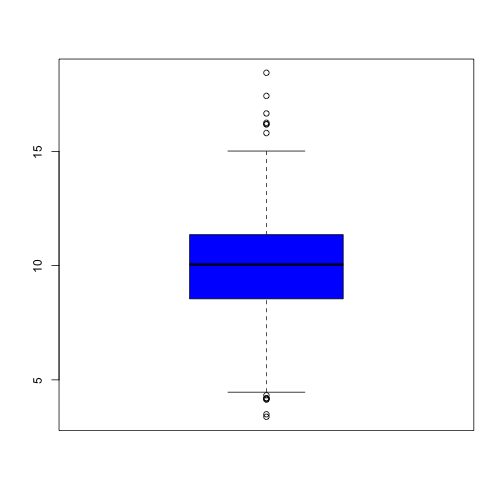### Histogram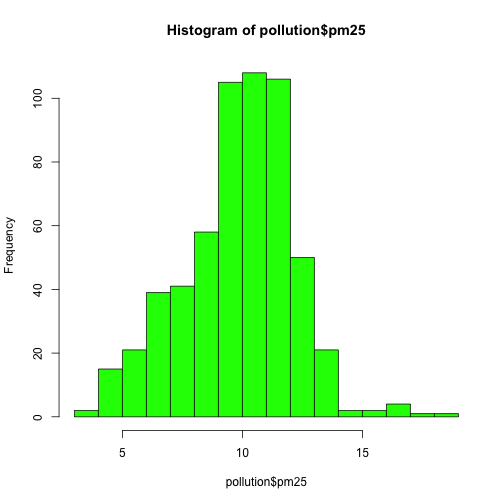### Histogram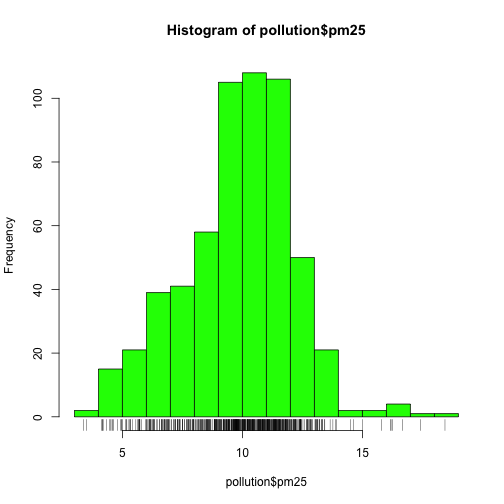### Histogram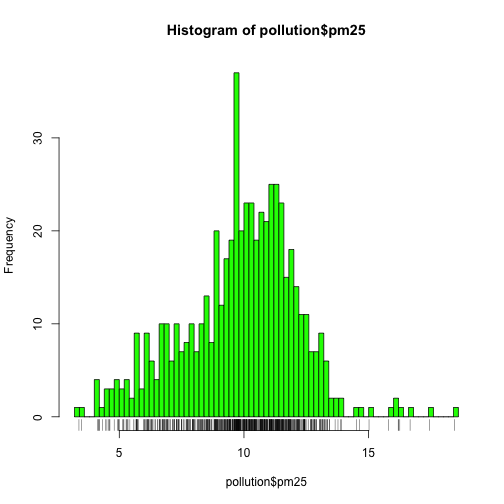boxplot(pollution$pm25) abline(h=12,col="blue")### Overlaying Features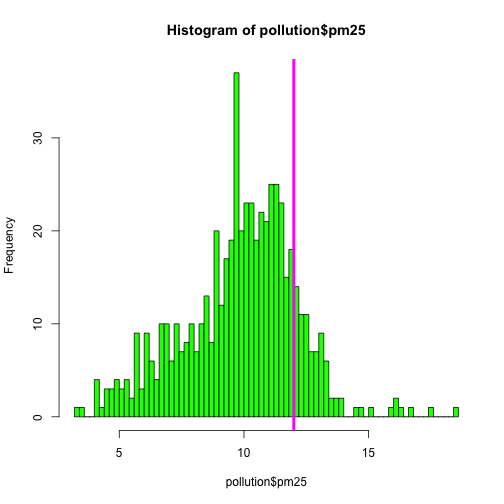### Barplot barplot(table(pollution$region), col = "wheat", main = "Number of Counties in Each Region")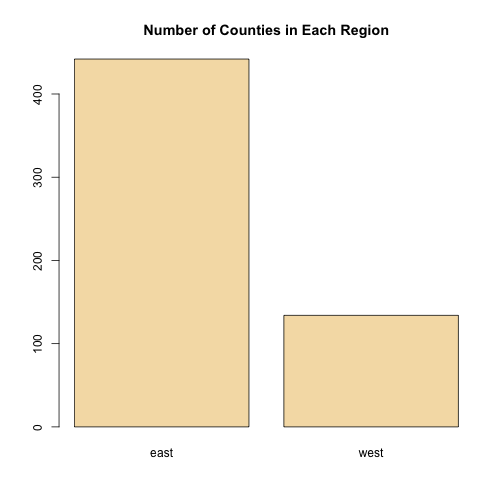# Two dimensions

• Multiple/overlayed 1-D plots (Lattice/ggplot2)
• Scatterplots
• Smooth scatterplots
• Overlayed/multiple 2-D plots; coplots
• Use color, size, shape to add dimensions
• Spinning plots
• Actual 3-D plots (not that useful)

### Multiple Boxplots

boxplot(pm25 ~ region, data = pollution, col = c("magenta","green"))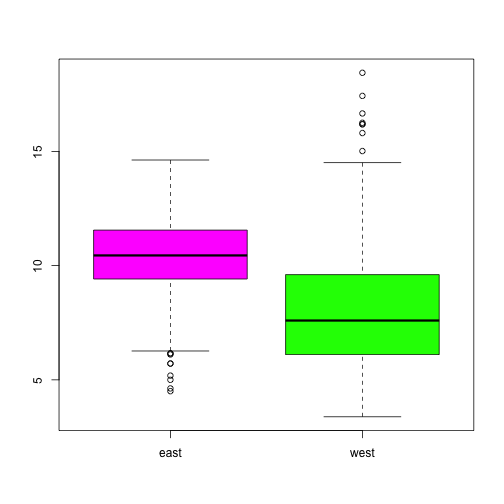### Multiple Histogram

par(mfrow = c(2, 1), mar = c(4, 4, 2, 1))
hist(subset(pollution, region == "east")$pm25, col = "magenta") hist(subset(pollution, region == "west")$pm25, col = "green")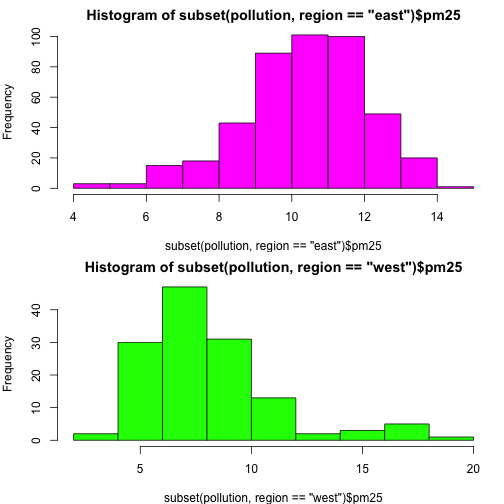### Scatterplot

with(pollution, plot(latitude, pm25))
abline(h=12,lwd=2,lty=2)### Scatterplot - Using color

with(pollution, plot(latitude, pm25,col=region))
abline(h=12,lwd=2,lty=2)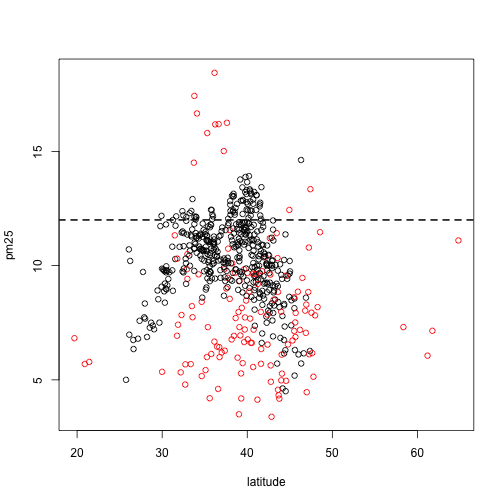### Multiple Scatterplot

par(mfrow = c(1, 2), mar = c(5, 4, 2, 1))
with(subset(pollution, region == "west"), plot(latitude, pm25, main = "West",col=region))
with(subset(pollution, region == "east"), plot(latitude, pm25, main = "East",col=region))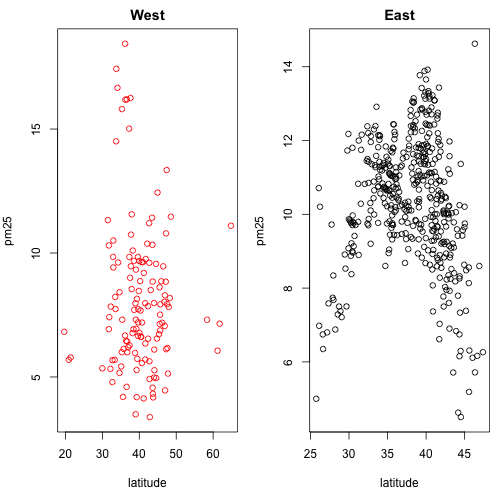### Summary

• Exploratory plots are “quick and dirty”
• Let you summarize the data (usually graphically) and highlight any broad features
• Explore basic questions and hypotheses (and perhaps rule them out)
• Suggest modeling strategies for the “next step”